Next: Angular momentum of a Up: Angular momentum Previous: Angular momentum of a

## Angular momentum of an extended object

Consider a rigid object rotating about some fixed axis with angular velocity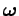. Let us model this object as a swarm ofparticles. Suppose that the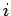th particle has mass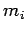, position vector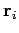, and velocity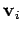. Incidentally, it is assumed that the object's axis of rotation passes through the origin of our coordinate system. The total angular momentum of the object,, is simply the vector sum of the angular momenta of theparticles from which it is made up. Hence,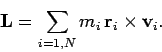(423)

Now, for a rigidly rotating object we can write (see Sect. 8.4)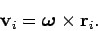(424)

Let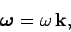(425)

where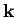is a unit vector pointing along the object's axis of rotation (in the sense given by the right-hand grip rule). It follows that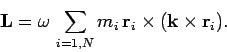(426)

Let us calculate the component ofalong the object's rotation axis--i.e., the component along theaxis. We can write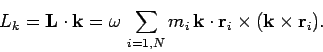(427)

However, since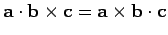, the above expression can be rewritten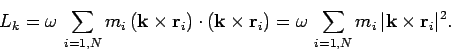(428)

Now,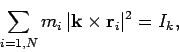(429)

where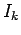is the moment of inertia of the object about theaxis. (see Sect. 8.6). Hence, it follows that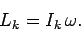(430)

According to the above formula, the component of a rigid body's angular momentum vector along its axis of rotation is simply the product of the body's moment of inertia about this axis and the body's angular velocity. Does this result imply that we can automatically write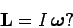(431)

Unfortunately, in general, the answer to the above question is no! This conclusion follows because the body may possess non-zero angular momentum components about axes perpendicular to its axis of rotation. Thus, in general, the angular momentum vector of a rotating body is not parallel to its angular velocity vector. This is a major difference from translational motion, where linear momentum is always found to be parallel to linear velocity.

For a rigid object rotating with angular velocity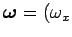,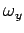,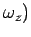, we can write the object's angular momentum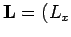,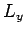,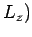in the form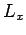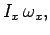(432)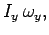(433)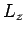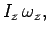(434)

where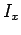is the moment of inertia of the object about the-axis, etc. Here, it is again assumed that the origin of our coordinate system lies on the object's axis of rotation. Note that the above equations are only valid when the-,-, and-axes are aligned in a certain very special manner--in fact, they must be aligned along the so-called principal axes of the object (these axes invariably coincide with the object's main symmetry axes). Note that it is always possible to find three, mutually perpendicular, principal axes of rotation which pass through a given point in a rigid body. Reconstructingfrom its components, we obtain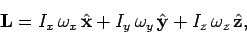(435)

whereis a unit vector pointing along the-axis, etc. It is clear, from the above equation, that the reasonis not generally parallel tois because the moments of inertia of a rigid object about its different possible axes of rotation are not generally the same. In other words, if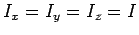then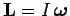, and the angular momentum and angular velocity vectors are always parallel. However, if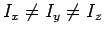, which is usually the case, thenis not, in general, parallel to.

Although Eq. (435) suggests that the angular momentum of a rigid object is not generally parallel to its angular velocity, this equation also implies that there are, at least, three special axes of rotation for which this is the case. Suppose, for instance, that the object rotates about the-axis, so that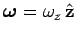. It follows from Eq. (435) that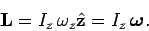(436)

Thus, in this case, the angular momentum vector is parallel to the angular velocity vector. The same can be said for rotation about the- or- axes. We conclude that when a rigid object rotates about one of its principal axes then its angular momentum is parallel to its angular velocity, but not, in general, otherwise.

How can we identify a principal axis of a rigid object? At the simplest level, a principal axis is one about which the object possesses axial symmetry. The required type of symmetry is illustrated in Fig. 86. Assuming that the object can be modeled as a swarm of particles--for every particle of mass, located a distancefrom the origin, and subtending an anglewith the rotation axis, there must be an identical particle located on diagrammatically the opposite side of the rotation axis. As shown in the diagram, the angular momentum vectors of such a matched pair of particles can be added together to form a resultant angular momentum vector which is parallel to the axis of rotation. Thus, if the object is composed entirely of matched particle pairs then its angular momentum vector must be parallel to its angular velocity vector. The generalization of this argument to deal with continuous objects is fairly straightforward. For instance, symmetry implies that any axis of rotation which passes through the centre of a uniform sphere is a principal axis of that object. Likewise, a perpendicular axis which passes through the centre of a uniform disk is a principal axis. Finally, a perpendicular axis which passes through the centre of a uniform rod is a principal axis.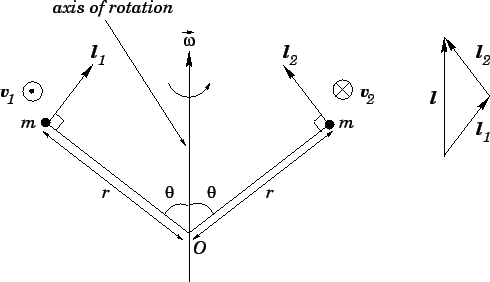Next: Angular momentum of a Up: Angular momentum Previous: Angular momentum of a
Richard Fitzpatrick 2006-02-02